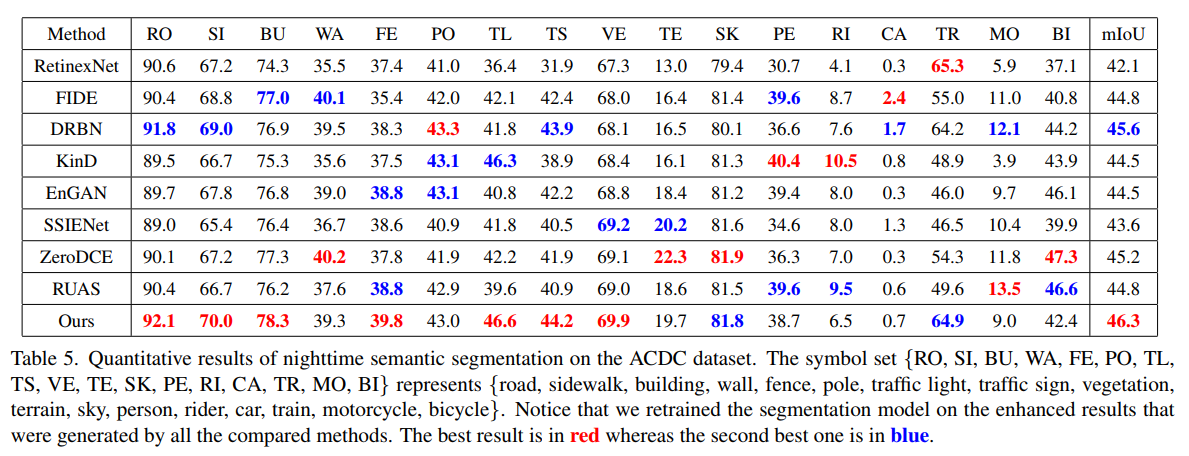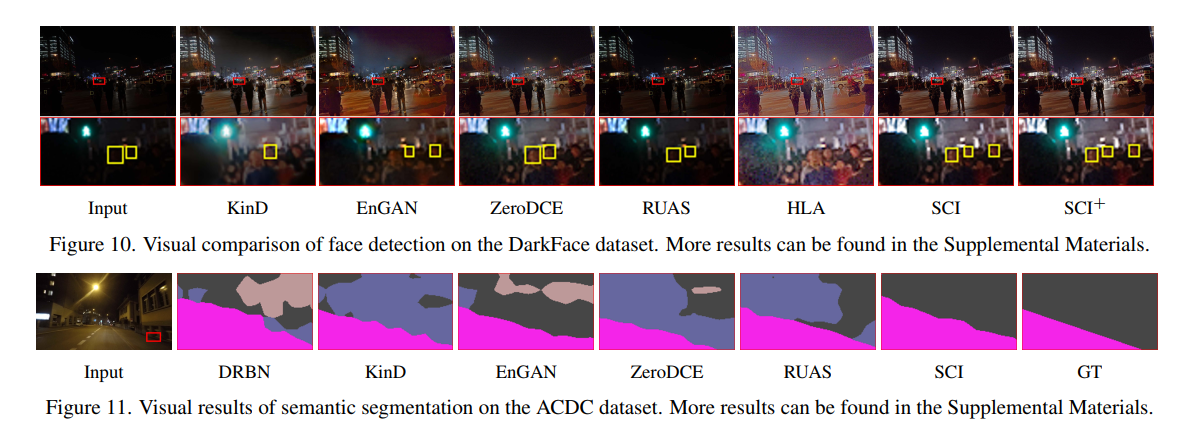> 文档中心 > 迈向快速、灵活、稳健的微光图像增强

# 迈向快速、灵活、稳健的微光图像增强

https://github.com/vis-opt-group/SCI。

# 1. 简介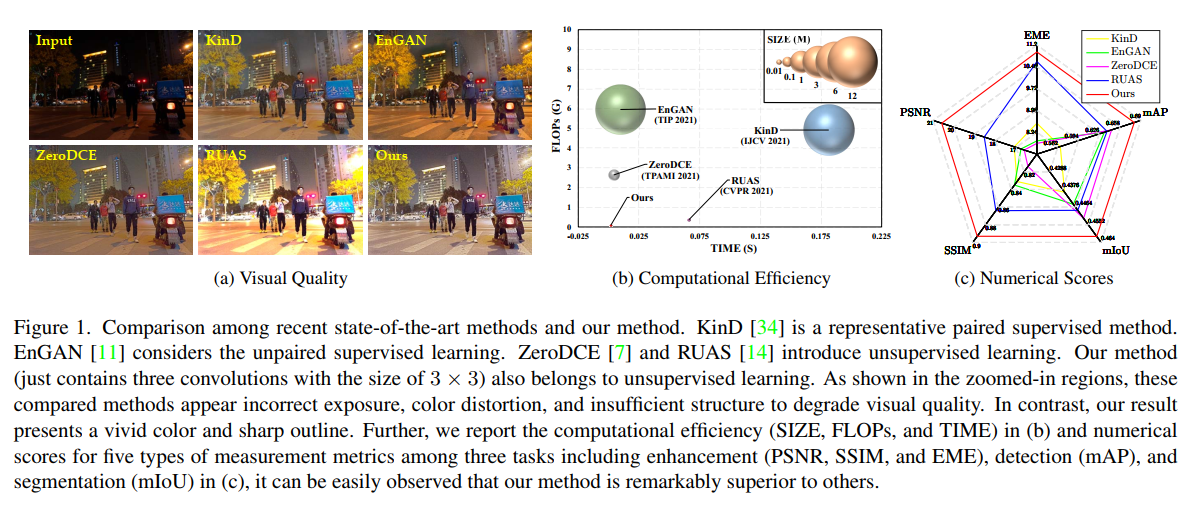• 我们开发了一个自校正的共享权重照明学习模块，使各阶段的结果收敛，提高了曝光稳定性，大大减少了计算量。据我们所知，这是第一个利用学习过程加速微光图像增强算法的工作。
• 我们定义了无监督训练损耗，在自校正模块的作用下约束各阶段的输出，赋予了对不同场景的适应能力。属性分析表明，SCI具有操作不敏感的适应性和模型无关的通用性，这是现有文献所没有的。
• 我们进行了大量的实验，以证明我们的方法优于其他最先进的方法。进一步在黑暗人脸检测和夜间语义分割方面的应用，揭示了我们的实用价值。简言之，SCI重新定义了基于网络的微光图像增强领域的视觉质量、计算效率和下游任务的性能的峰值点。

# 2. 该方法

## 2.1. 照明学习与权重共享

F ( x t ) : { u t= H θ ( x t ), x 0= y x t + 1 = x t+ u t (1) \mathcal{F}\left(\mathbf{x}^{t}\right):\left\{\begin{array}{l} \mathbf{u}^{t}=\mathcal{H}_{\boldsymbol{\theta}}\left(\mathbf{x}^{t}\right), \mathbf{x}^{0}=\mathbf{y} \\ \mathbf{x}^{t+1}=\mathbf{x}^{t}+\mathbf{u}^{t} \end{array}\right. \tag{1}

## 2.2. Self-Calibrated模块

G ( x t ) : { z t= y ⊘ x t s t= K ϑ ( z t ) v t= y + s t (2) \mathcal{G}\left(\mathbf{x}^{t}\right):\left\{\begin{array}{l} \mathbf{z}^{t}=\mathbf{y} \oslash \mathbf{x}^{t} \\ \mathbf{s}^{t}=\mathcal{K}_{\vartheta}\left(\mathbf{z}^{t}\right) \\ \mathbf{v}^{t}=\mathbf{y}+\mathbf{s}^{t} \end{array}\right. \tag{2}

F ( x t ) → F ( G ( x t ) ) (3) \mathcal{F}\left(\mathbf{x}^{t}\right) \rightarrow \mathcal{F}\left(\mathcal{G}\left(\mathbf{x}^{t}\right)\right) \tag{3}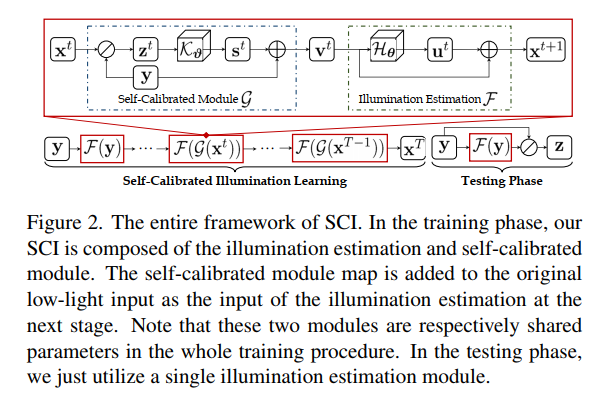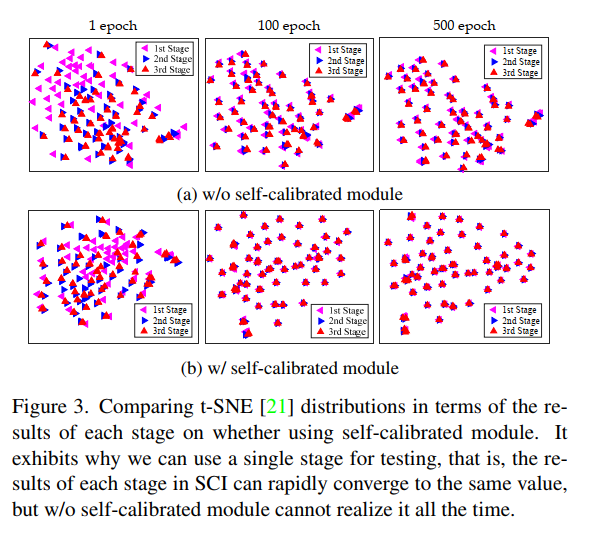## 2.3. 非监督训练的损失

L f = ∑ t = 1 T ∥ x t − ( y + st−1 ) ∥ 2 (4) \mathcal{L}_{f}=\sum_{t=1}^{T}\left\|\mathbf{x}^{t}-\left(\mathbf{y}+\mathbf{s}^{t-1}\right)\right\|^{2} \tag{4}

L s = ∑ i = 1 N ∑ j ∈ N ( i ) w i , j ∣ x i t − x j t ∣ (5) \mathcal{L}_{s}=\sum_{i=1}^{N} \sum_{j \in \mathcal{N}(i)} w_{i, j}\left|\mathbf{x}_{i}^{t}-\mathbf{x}_{j}^{t}\right| \tag{5}

# 3.属性探索算法

## 3.1. Operation-Insensitive适应性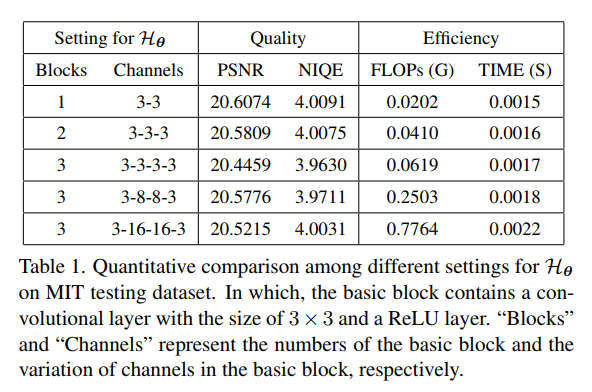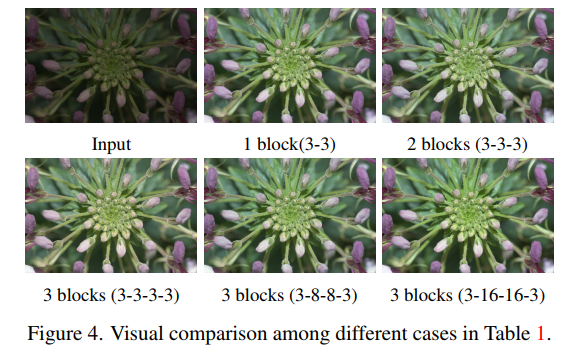## 3.2. Model-Irrelevant普遍性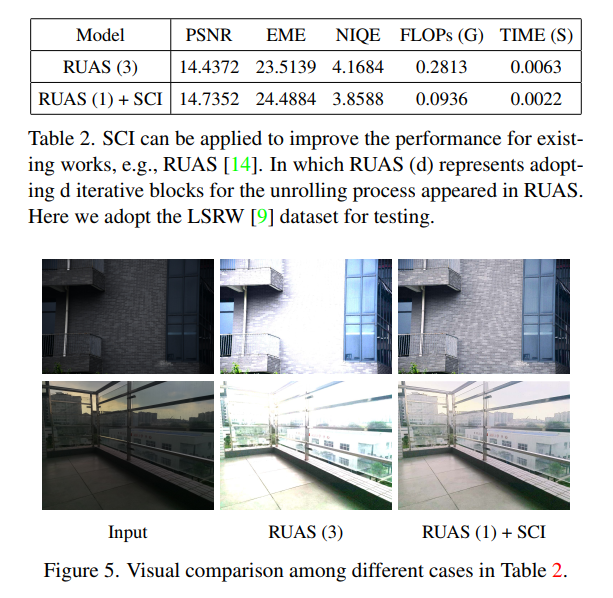# 4. 实验结果

## 4.2. 基准实验评价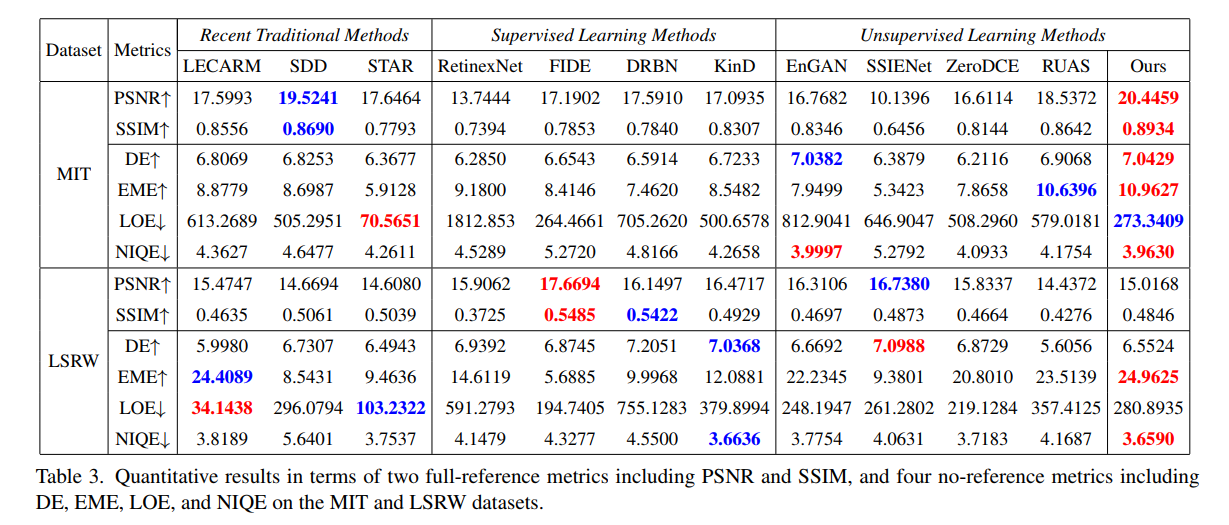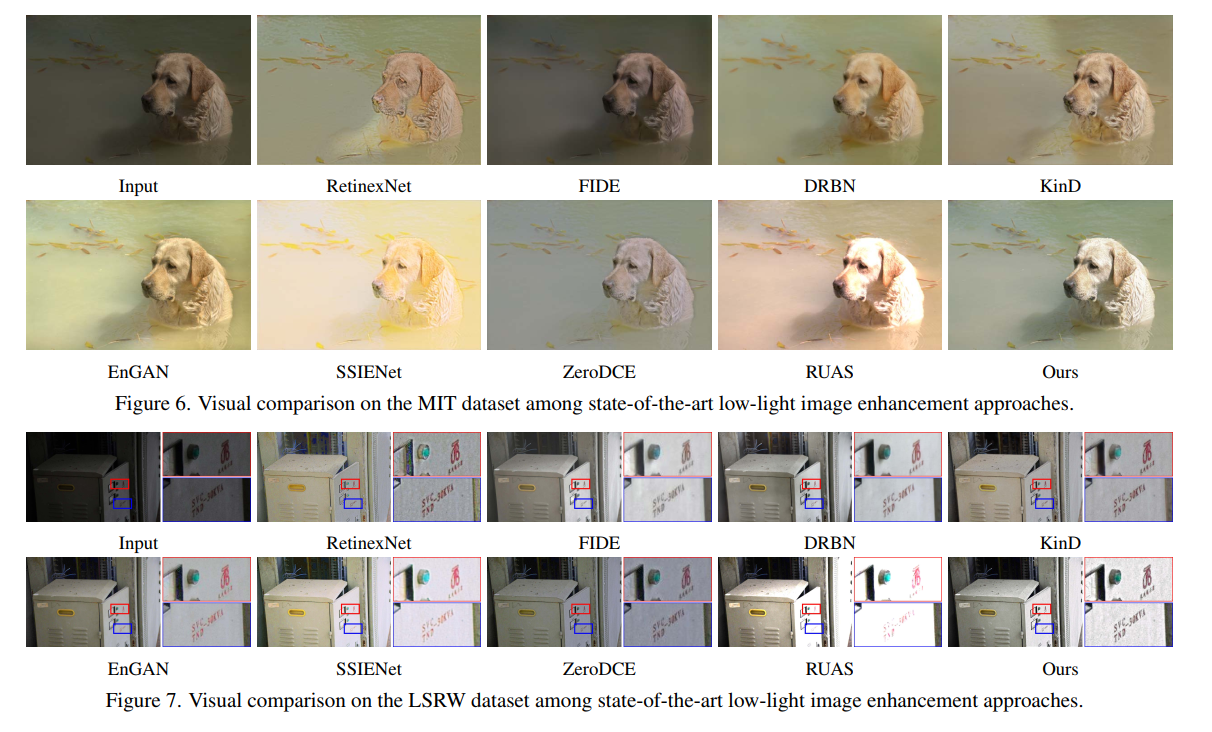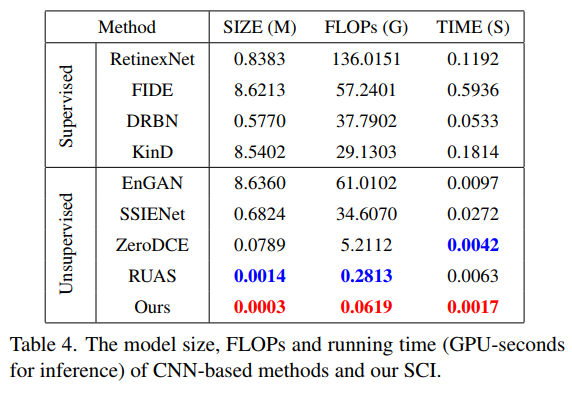## 4.3. 在野外实验评价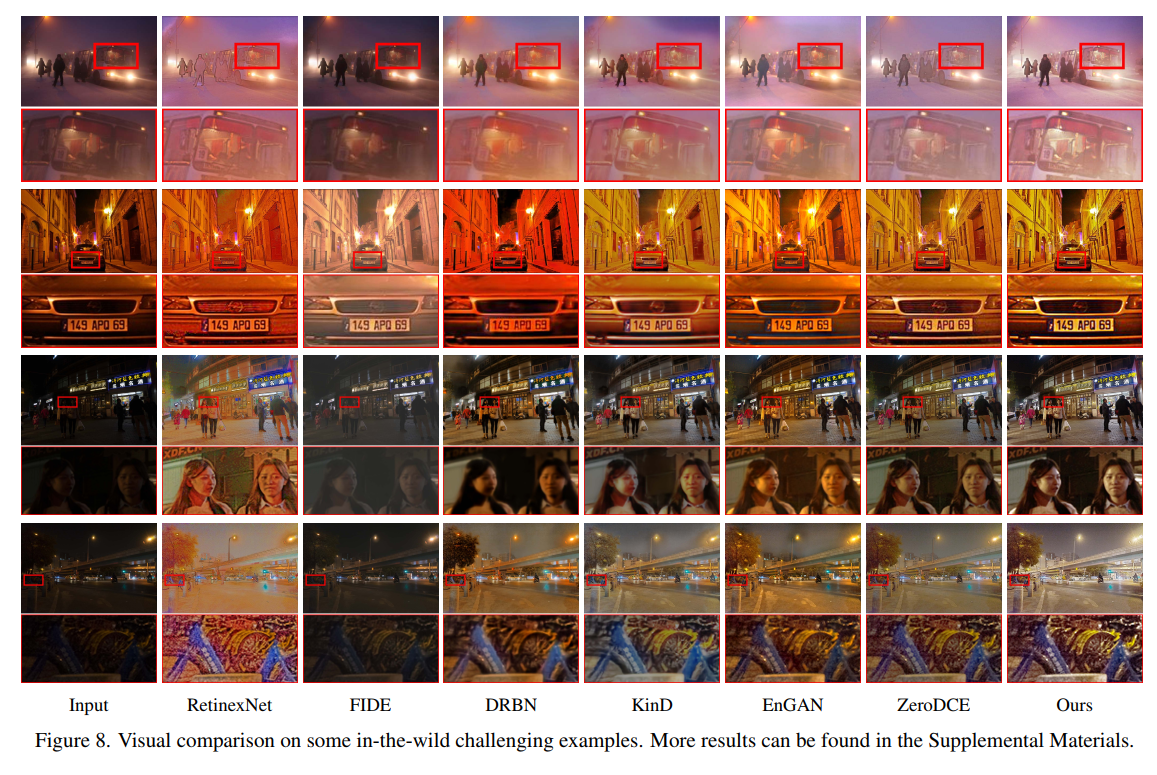## 4.4. 黑暗的人脸检测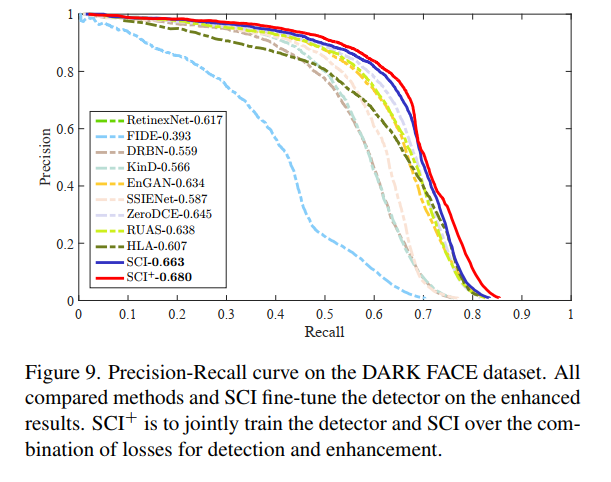## 4.5. 夜间的语义分割